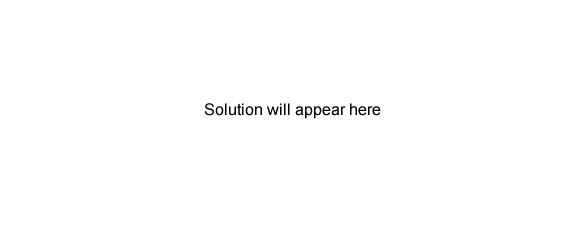Volumetric analysis exercises (2008 VCE) 0.415 g of a pure acid, H2X(s), is added to exactly 100 mL of 0.105 M NaOH(aq). A reaction occurs according to the equation H2X(s) + 2NaOH(aq) → Na2X(aq) + 2H2O(l) The NaOH is in excess. This excess NaOH requires 25.21 mL of 0.197 M HCl(aq) for neutralisation. Calculate i. the amount, in mol, of NaOH that is added to the acid H2X initially. Solution ii. the amount, in mol, of NaOH that reacts with the acid H2X Solution. iii. the molar mass, in g/mol, of the acid H2X. Solution